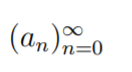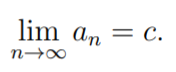# Constant Sequence, Eventually Constant

A sequence where all the terms are the same real number is a constant sequence. For example, the sequence {4} = (4, 4, 4, …) is a constant sequence.

More formally, we can write a constant sequence as an = c for all n, where an are the terms of the series and c is the constant. The terms of the sequence {5} could be written as:

• a1 = 5
• a2 = 5
• a3 = 5
• a4 = 5
• an = 5

The constant sequence is a special case of the eventually constant sequence, where a natural number N exists, so that if n ≥ N then an = aN. In other words, somewhere along the line, the sequence will converge on a natural number. For example, the sequence {1, 2, 3, 4, 4, 4, 4, 4, …} is eventually constant.

## Constant Sequence Rule

The constant sequence rule states that the limit of an infinite constant sequence with value “c” is also “c”. More formally, we can write that as :

If a sequence is defined bywith constant value “c”, then:## Constant Sequence Convergence

All constant sequences converge to the real-numbered term in the sequence . For example, the sequence (2, 2, 2, …) converges to 2.

The proof for convergence of a constant sequence is fairly straightforward and short, because you’re only dealing with a single constant, c.

Suppose that an = c for all n ∈ ℕ. To prove the limit for the sequence, as n → ∞, is c, you need to show that, given any ε > 0, there
is a natural number (n N ∈ ℕ) where n ≥ N implies |an − c| < ε.

We know that an = c for n ∈ ℕ, so we have |an – c| = 0 for all n ∈ ℕ. This means that the statement above (n ≥ N implies |an − c| < ε) holds for all ε > 0 and all n ∈ ℕ.

## References

 Math 8 Winter 2020 Taylor Polynomials and Taylor Series Day 2. Retrieved April 3, 2021 from: https://math.dartmouth.edu/~m8w20/lectures/m8w20notes2.pdf
 6.4. Properties of Limits. Retrieved April 4, 2021 from: http://people.reed.edu/~mayer/math111.html/header/node34.html
 Hildebrand, A. Math 347 Worksheet: Epsilonics, I: Sequences and Limits —SOLUTIONS. Retrieved April 4, 2021 from: https://faculty.math.illinois.edu/~hildebr/347.summer19/epsilonics1sol.pdf

CITE THIS AS:
Stephanie Glen. "Constant Sequence, Eventually Constant" From StatisticsHowTo.com: Elementary Statistics for the rest of us! https://www.statisticshowto.com/constant-sequence/